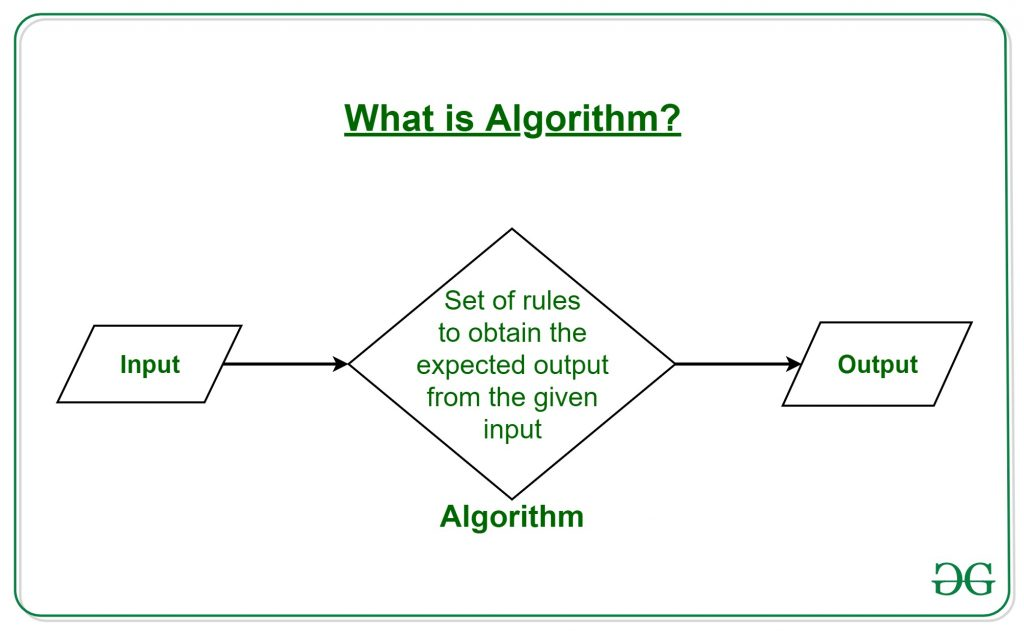# Algorithms

• Last Updated : 26 Jul, 2023

## What is an Algorithm?

The word Algorithm means “A set of rules to be followed in calculations or other problem-solving operations” Or “A procedure for solving a mathematical problem in a finite number of steps that frequently involves recursive operations “.

Therefore Algorithm refers to a sequence of finite steps to solve a particular problem.

Algorithms can be simple and complex depending on what you want to achieve.Introduction to Algorithms:

## Types of Algorithms:

There are several types of algorithms available. Some important algorithms are:

1. Brute Force Algorithm: It is the simplest approach for a problem. A brute force algorithm is the first approach that comes to finding when we see a problem.

2. Recursive Algorithm: A recursive algorithm is based on recursion. In this case, a problem is broken into several sub-parts and called the same function again and again.

3. Backtracking Algorithm: The backtracking algorithm basically builds the solution by searching among all possible solutions. Using this algorithm, we keep on building the solution following criteria. Whenever a solution fails we trace back to the failure point and build on the next solution and continue this process till we find the solution or all possible solutions are looked after.

4. Searching Algorithm: Searching algorithms are the ones that are used for searching elements or groups of elements from a particular data structure. They can be of different types based on their approach or the data structure in which the element should be found.

5. Sorting Algorithm: Sorting is arranging a group of data in a particular manner according to the requirement. The algorithms which help in performing this function are called sorting algorithms. Generally sorting algorithms are used to sort groups of data in an increasing or decreasing manner.

6. Hashing Algorithm: Hashing algorithms work similarly to the searching algorithm. But they contain an index with a key ID. In hashing, a key is assigned to specific data.

7. Divide and Conquer Algorithm: This algorithm breaks a problem into sub-problems, solves a single sub-problem and merges the solutions together to get the final solution. It consists of the following three steps:

• Divide
• Solve
• Combine

8. Greedy Algorithm: In this type of algorithm the solution is built part by part. The solution of the next part is built based on the immediate benefit of the next part. The one solution giving the most benefit will be chosen as the solution for the next part.

9. Dynamic Programming Algorithm: This algorithm uses the concept of using the already found solution to avoid repetitive calculation of the same part of the problem. It divides the problem into smaller overlapping subproblems and solves them.

10. Randomized Algorithm: In the randomized algorithm we use a random number so it gives immediate benefit. The random number helps in deciding the expected outcome.

### Topics:

Analysis of Algorithms:

Searching and Sorting:

Greedy Algorithms:

Dynamic Programming:

Pattern Searching:

Backtracking:

Divide and Conquer:

Geometric Algorithm:

Mathematical Algorithms:

Bitwise Algorithms:

Graph Algorithms:

Randomized Algorithms:

Branch and Bound:

Quizzes:

Please see Data Structures and Advanced Data Structures for Graph, Binary Tree, BST and Linked List based algorithms.